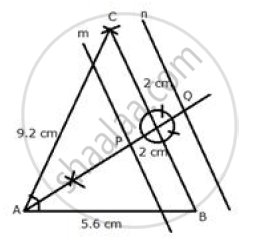# Construct a Triangle Abc, with Ab = 5.6 Cm, Ac = Bc = 9.2 Cm. Find the Points Equidistant from Ab and Ac; and Also 2 Cm from Bc. Measure the Distance Between the Two Points Obtained. - Mathematics

Sum

Construct a triangle ABC, with AB = 5.6 cm, AC = BC = 9.2 cm. Find the points equidistant from AB and AC; and also 2 cm from BC. Measure the distance between the two points obtained.

#### SolutionSteps of Construction:
i) Draw a line segment AB = 5.6 cm
ii) From A and B, as centers and radius 9.2 cm, make two arcs which intersect each other at C.
iii) Join CA and CB.
iv) Draw two lines n and m parallel to BC at a distance of 2 cm
v) Draw the angle bisector of ∠BAC which intersects m and n at P and Q respectively.
P and Q are the required points which are equidistant from AB and AC.
On measuring the distance between P and Q is 4.3 cm.

Concept: Constructions Under Loci
Is there an error in this question or solution?

#### APPEARS IN

Selina Concise Maths Class 10 ICSE
Chapter 16 Loci (Locus and Its Constructions)
Exercise 16 (B) | Q 23 | Page 241